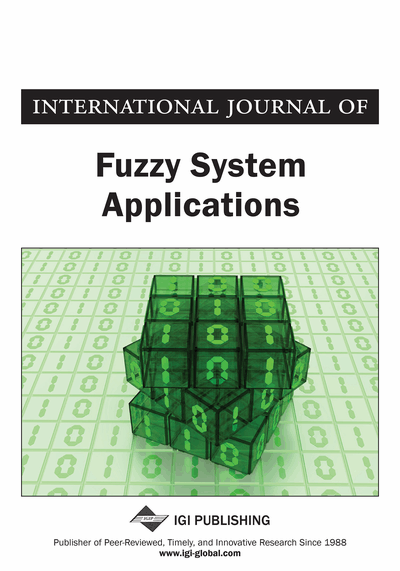# Ordered Intuitionistic Fuzzy Soft Sets and Its Application in Decision Making Problem

Pachaiyappan Muthukumar, Sai Sundara Krishnan Gangadharan
DOI: 10.4018/IJFSA.2018070105
OnDemand:
(Individual Articles)
Available
\$37.50
No Current Special Offers

## Abstract

In this article, some new basic operations and results of Ordered Intuitionistic Fuzzy Soft (OIFS) sets, such as equality, complement, subset, union, intersection, OR, and AND operators along with several examples are investigated. Further, based on the analysis of several operations on OIFS sets, numerous algebraic properties and famous De Morgans inclusions and De Morgans laws are established. Finally, using the notions of OIFS sets, an algorithm is developed and implemented in a numerical example.
Article Preview
Top

## 1. Introduction

Molodtsov (1999, 2004) initiated the concept of soft set theory as a new mathematical tool for dealing with uncertainties which are free from these constraints. Maji et al. (2003) have done research on soft set theory and they defined the operations of AND, OR, union, intersection, and complement. Maji et al. (2001) introduced some properties regarding fuzzy soft union, intersection, complement, and DeMorgan's law. These results are further revised and improved by Ahmad and Kharal (2009). Maji et al. (2001, 2004) has developed the concept of Intuitionistic Fuzzy Soft (IFS) sets using Intuitionistic Fuzzy (IF) sets introduced by Atanassov (1986).

Yang et al. (2007, 2009) extended this theory to fuzzy soft set theory and discussed some of its applications. Further, Kharal and Ahmad (2009) introduced the notion of a mapping on the classes of fuzzy soft sets which is a vital idea of the advanced development of any innovative area of the mathematical sciences. Kong et al. (2009) mentioned on a fuzzy soft set theoretic approach to decision making problems. Xiao et al. (2009) discussed a combined forecasting approach based on the fuzzy soft sets. Majumdar and Samanta (2010) gave an idea of soft mappings and some of their properties. Further, an application of soft mapping in medical diagnosis has been shown. Majumdar et al. (2011) established generalized fuzzy soft sets and proposed a new student ranking system based on this theory. Cagman et al. (2010, 2012, 2013) initiated the notion of soft groups, soft matrix theory, Fuzzy Soft Matrix, Fuzzy parameterized fuzzy soft set and uni-int decision making problems in soft set theory. Further, they defined four types of distances between two IFSSs and proposed similarity measures of two IFSSs. Also construct a decision method which is applied to a medical diagnosis problem that is based on similarity measures of IFSSs. Majumdar et al. (2008, 2010) established similarity measure between two generalized fuzzy soft sets and applied in the medical diagnosis problem.

## Complete Article List

Search this Journal:
Reset
Volume 13: 1 Issue (2024)
Volume 12: 1 Issue (2023)
Volume 11: 4 Issues (2022)
Volume 10: 4 Issues (2021)
Volume 9: 4 Issues (2020)
Volume 8: 4 Issues (2019)
Volume 7: 4 Issues (2018)
Volume 6: 4 Issues (2017)
Volume 5: 4 Issues (2016)
Volume 4: 4 Issues (2015)
Volume 3: 4 Issues (2013)
Volume 2: 4 Issues (2012)
Volume 1: 4 Issues (2011)
View Complete Journal Contents Listing